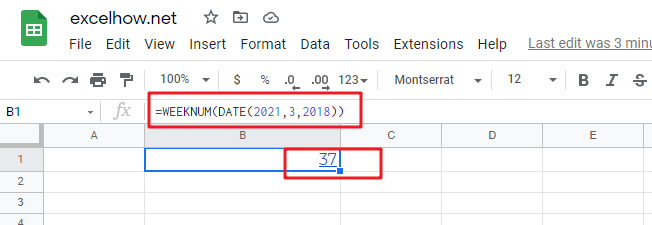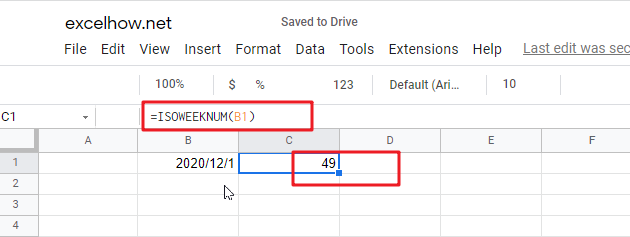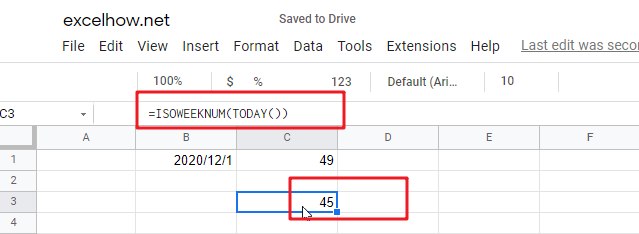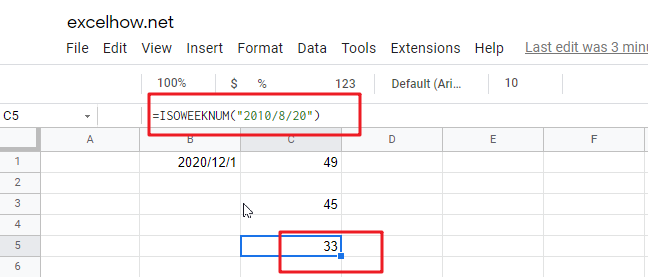This post will guide you how to use Google Sheets WEEKNUM function with syntax and examples.

## Description

The Google Sheets WEEKNUM function returns the week number of a specific date, and the returned value is ranging from 1 to 53. And this function will start to count the week number from January 1 and the Sunday is the first day in one week by default.

The WEEKNUM function can be used to get a number representing the week of the year where the provided date falls in google sheets. The purpose of this function is to get the week number for a given date and it will return a number between 1 and 54.

The WEEKNUM function is a build-in function in Google Sheets and it is categorized as a Date function.

## Syntax

The syntax of the WEEKNUM function is as below:

=WEEKNUM (serial_number,[return_type])

Where the WEEKNUM function arguments is:

• Serial_numberThis is a required argument. A date that you want to get the week number. The dates should be typed by the DATE function to make sure it is a valid date. For example, use DATE(2021,3,20) to pass into the WEEKNUM function and then it will return the week number.
• return_typeThis is an optional argument. A number that determines the first day of the week for the year, the default value is 1.
 Return_type Week begins on System 1 or omitted Sunday 1 2 Monday 1 11 Monday 1 12 Tuesday 1 13 Wednesday 1 14 Thursday 1 15 Friday 1 16 Saturday 1 17 Sunday 1 21 Monday 2

Note:

• If serial_number is out of range for the current date base value, a #NUM! Error is returned.
• If return_type is out of the range specified in the table above, a #NUM! Error is returned.
• A serial date is how the google sheets stores dates and it represents the number of days since 1900-01-01, so the January 1, 1900 date is serial number 1 by default.

## Google Sheets WEEKNUM Function Examples

The below examples will show you how to use google sheets WEEKNUM Function to return the week number from a date.

1# get number of the week from a date value 2021/03/20, enter the following formula in Cell B1.

`=WEEKNUM(DATE(2021,3,2018))`This post will guide you how to use Google Sheets ISOWEEKNUM function with syntax and examples.

## Description

The Google Sheets ISOWEEKNUM returns the number of the ISO week of the year for a given date. ISO weeks begin on Monday and number 1 is the first week in that year.

The ISOWEEKNUM function can be used to get a week number from 1 to 54 that follows ISO standards in google sheets. The purpose of this function is to get ISO week number for a given data and its returned values is a number between 1 and 54.

The ISOWEEKNUM function is a build-in function in Google Sheets and it is categorized as a Date function.

## Syntax

The syntax of the ISOWEEKNUM function is as below:

=ISOWEEKNUM (date)

Where the ISOWEEKNUM function arguments is:

• Date -This is a required argument. The date for which to calculate the ISO week number. It must be a cell reference that containing a date value, number or a function that returning a date type in google sheets.

Note:

• You can use the DATE function to enter into a date value, or it will be return errors for a text values.
• If the `date` argument is not a valid date type, ISOWEEKNUM function returns the #VALUE! Error message.
• You need to know the WEEKNUM function will also start week number 1 on the first day of the year, then increment week numbers on Sundays.

## Google Sheets ISOWEEKNUM Function Examples

The below examples will show you how to use google sheets ISOWEEKNUM Function to get the ISO week number for a given date.

1# calculate the ISO week number for a given date in Cell B1, using the following formula:

`=ISOWEEKNUM(B1)`2# calculate the ISO WEEK number of current date

If you want to get the ISO week number for the current date in google sheets, and you can use the ISOWEEKNUM formula in combination with the TODAY function, just using the following formula:

`=ISOWEEKNUM(TODAY())`3# calculate the ISO WEEK number with a date text string

You can enter a date text string into ISOWEEKNUM function instead of a cell reference that contain a date type value, just see the below formula:

`=ISOWEEKNUM("2010/8/20")`